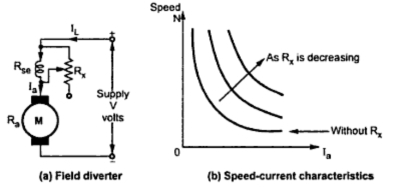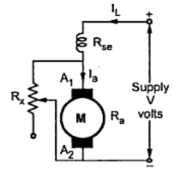### Speed Control of D.C. Series Motor : Flux Control

Speed Control of D.C. Series Motor (Part1)
The flux produced by the winding depends on the m.m.f. i.e. magnetomotive force which is the product of current and the number of turns of the winding through which current is passing. So flux can be changed either by changing the current by adding a resistance or by changing the number of turns of the winding. Let us study the various methods based on this principle.

1. Flux Control
The various methods of flux control in a d.c. series motor are explained below :
1.1 Field Divertor Mehtod
In this method the series field winding is shunted by a variable resistance () known as field divertor. The arrangement is shown in the Fig. 1(a).
Due to the parallel path of Rx , by adjusting the value of Rx, any amount of current can be diverted through the divertor. Hence current through the field winding can be adjusted as per the requirement. Due to this, the flux gets controlled and hence the speed of the motor gets controlled.
By this method the speed of the motor can be controlled above rated value. The speed armature current characteristics with range in Rx is shown in the Fig. 1(b).Fig. 1

1.2 Armature Divertor Method
This method is used for the motor which require constant load torque. An armature of the motor is shunted with an external variable resistance (Rx) as shown in the Fig. 2. This resistance Rx is called armature divertor.Fig. 2  Armature divertor
Any amount of armature current can be diverted through the divertor. Due to this , armature current reduces. But as T α Φ Ia and load torque is constant, the flux is to be increased. So current through field winding increases, so flux increases and speed of the motor reduces. The method is used to control the speed below the normal value.
1.3 Tapped Field Method
In this method, flux change is achieved by changing the number of turns of the field winding. The field winding is provided with the taps as shown in the Fig. 3.Fig. 3  Tapped field
The selector switch 'S' is provided to select the number of turns (taps) as per the requirement. When the switch 'S' is in position 1 the entire filed winding is in the circuit and motor runs with normal speed. As switch is moved from position 1 to 2 and onwards, the number of turns of the field winding in the circuit decreases. Due to this m.m.f. require to produce the flux, decreases. Due to this flux produced decreases, increasing the speed of the motor above rated value. The method is often used in electric traction.
1.4 Series - Parallel Connection of Field
In this method, the field coil is divided into various parts. These parts can then be connected in series or parallel as per the requirement. The Fig. 4(a) and (b) show the two parts of field coil connected in series and parallel.Fig. 4  Series parallel grouping of field coils

For the same torque, if the field coil is arranged in series or parallel, m.m.f. produced by the coils changes, hence the flux produced also changes. Hence speed can be controlled. Some fixed speeds only can be obtained by parallel grouping, the m.m.f. produced decreases, hence higher speed can be obtained by parallel grouping. The method is generally used in case of fan motors.